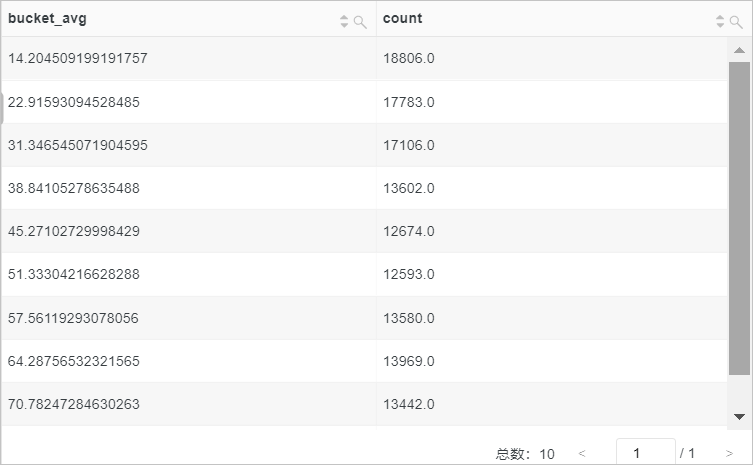This topic describes the syntax of approximate functions. This topic also provides examples on how to use the functions.

The following table describes the approximate functions that are supported by Log Service.

Notice If you want to use strings in analytic statements, you must enclose strings in single quotation marks (''). Strings that are not enclosed or enclosed in double quotation marks ("") indicate field names or column names. For example, 'status' indicates the status string, and status or "status" indicates the status log field.
Function Syntax Description
approx_distinct function approx_distinct(x) Estimates the number of unique values in x. The default standard error is 2.3%.
approx_distinct(x, e) Estimates the number of unique values in x. You can specify a custom standard error.
approx_percentile function approx_percentile(x, percentage) Lists the values of x in ascending order and returns the x value that is approximately at the percentage position.
approx_percentile(x, array[percentage01, percentage02...]) Lists the values of x in ascending order and returns the x values that are approximately at the percentage01 and percentage02 positions.
approx_percentile(x, weight, percentage) Calculates the product of each x value and the weight of the value, sorts all values in ascending order of the calculated products, and then returns the x value that is approximately at the percentage position.
approx_percentile(x, weight, array[percentage01, percentage02...]) Calculates the product of each x value and the weight of the value, sorts all values in ascending order of the calculated products, and then returns the x values that are approximately at the percentage01 and percentage02 positions.
approx_percentile(x, weight, percentage, accuracy) Calculates the product of each x value and the weight of the value, sorts all values in ascending order of the calculated products, and then returns the x value that is approximately at the percentage position. You can specify the accuracy of the return value.
numeric_histogram function numeric_histogram(bucket, x) Computes the approximate histogram of x based on the number of histogram columns. The number is specified in the bucket parameter. The return value is of the JSON type.
numeric_histogram(bucket, x, weight) Computes the approximate histogram of x based on the number of histogram columns. The number is specified in the bucket parameter. The return value is of the JSON type. You can specify weights for the values of x.
numeric_histogram_u function numeric_histogram_u(bucket, x) Computes the approximate histogram of x based on the number of histogram columns. The number is specified in the bucket parameter. A table that contains multiple rows and columns is returned.

## approx_distinct function

The approx_distinct function is used to estimate the number of unique values in x.

### Syntax

• An approx_distinct function of the following syntax is used to estimate the number of unique values in x. The default standard error is 2.3%.
``approx_distinct(x)``
• An approx_distinct function of the following syntax is used to estimate the number of unique values in x. You can specify a custom standard error.
``approx_distinct(x, e)``

### Parameters

Parameter Description
x The value of this parameter is of an arbitrary data type.
e The custom standard error. Valid values: 0.0115 to 0.26.

The bigint type.

### Examples

• Example 1: Use the count function to calculate the number of page views (PVs). Then, use the approx_distinct function to estimate the unique values of the client_ip field as the number of unique visitors (UVs). The standard error is 2.3%.
• Query statement
``* |SELECT count(*) AS PV, approx_distinct(client_ip) AS UV``
• Query and analysis result• Example 2: Use the count function to calculate the number of PVs. Then, use the approx_distinct function to estimate the unique values of the client_ip field as the number of UVs. The standard error is 10%.
• Query statement
``* |SELECT count(*) AS PV, approx_distinct(client_ip,0.1) AS UV``
• Query and analysis result## approx_percentile function

The approx_percentile function is used to list the values of x in ascending order and return the value that is approximately at the percentage position.

### Syntax

• An approx_percentile function of the following syntax is used to list the values of x in ascending order and return the x value that is approximately at the percentage position. The return value is of the double type.
``approx_percentile(x, percentage)``
• An approx_percentile function of the following syntax is used to list the values of x in ascending order and return the x values that are approximately at the percentage01 and percentage02 positions. The return value is of the array(double,double) type.
``approx_percentile(x, array[percentage01, percentage02...])``
• An approx_percentile function of the following syntax is used to calculate the product of each x value and the weight of the value, sort all values in ascending order of the calculated products, and then return the x value that is approximately at the percentage position. The return value is of the double type.
``approx_percentile(x, weight, percentage)``
• An approx_percentile function of the following syntax is used to calculate the product of each x value and the weight of the value, sort all values in ascending order of the calculated products, and then return the x values that are approximately at the percentage01 and percentage 02 positions. The return value is of the array(double,double) type.
``approx_percentile(x, weight, array[percentage01, percentage02...])``
• An approx_percentile function of the following syntax is used to calculate the product of each x value and the weight of the value, sort all values in ascending order of the calculated products, and then return the x value that is approximately at the percentage position. The return value is of the double type. You can specify the accuracy of the return value.
``approx_percentile(x, weight, percentage, accuracy)``

### Parameters

Parameter Description
x The value of this parameter is of the double type.
percentage The percentage value. Value range: [0, 1].
accuracy The accuracy. Value range: (0, 1).
weight The weight. A weight must be an integer that is greater than 1.

After you specify weights, the system calculates the product of each x value and the weight of the value and sorts all values in ascending order of the calculated products.

### Return value type

The double or array(double,double) type.

### Examples

• Example 1: Sort the values of the request_time column in ascending order, and then return the value that is approximately at the 50% position in the request_time field.
• Query statement
``*| SELECT approx_percentile(request_time,0.5)``
• Query and analysis result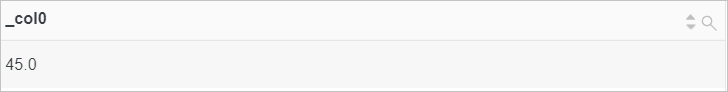• Example 2: Sort the values of the request_time column in ascending order, and then return the values that are approximately at the 10%, 20%, and 70% positions in the request_time field.
• Query statement
``*| SELECT approx_percentile(request_time,array[0.1,0.2,0.7])``
• Query and analysis result• Example 3: Calculate the product of each request_time value and the weight of the value, sort all request_time values in ascending order of the calculated products, and then return the value that is approximately at the 50% position in the request_time field. If the value of request_time is less than 20, the weight is 100. Otherwise, the weight is 10.
• Query statement
``````* |
SELECT
approx_percentile(
request_time,case
when request_time < 20 then 100
else 10
end,
0.5
)``````
• Query and analysis result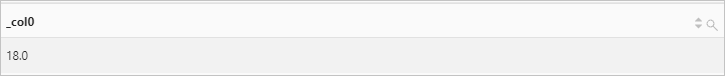• Example 4: Calculate the product of each request_time value and the weight of the value, sort all request_time values in ascending order of the calculated products, and then return the values that are approximately at the 80% and 90% positions in the request_time field. If the value of request_time is less than 20, the weight is 100. Otherwise, the weight is 10.
• Query statement
``````* |
SELECT
approx_percentile(
request_time,case
when request_time < 20 then 100
else 10
end,
array [0.8,0.9]
)``````
• Query and analysis result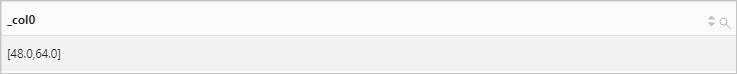• Example 5: Calculate the product of each request_time value and the weight of the value, sort all request_time values in ascending order of the calculated products, and then return the value that is approximately at the 50% position in the request_time field. The accuracy is 0.2. If the value of request_time is less than 20, the weight is 100. Otherwise, the weight is 10.
• Query statement
``````* |
SELECT
approx_percentile(
request_time,case
when request_time < 20 then 100
else 10
end,
0.5,
0.2
)``````
• Query and analysis result## numeric_histogram function

The numeric_histogram function is used to compute the approximate histogram of x based on the number of histogram columns. The number is specified in the bucket parameter. The return value is of the JSON type.

### Syntax

• A numeric_histogram function of the following syntax is used to compute the approximate histogram of x based on the number of histogram columns. The number is specified in the bucket parameter.
``numeric_histogram(bucket, x)``
• A numeric_histogram function of the following syntax is used to compute the approximate histogram of x based on the number of histogram columns. The number is specified in the bucket parameter. You can specify weights for the values of x.
``numeric_histogram(bucket, x, weight)``

### Parameters

Parameter Description
bucket The number of columns in the histogram. The value of this parameter is of the bigint type.
x The value of this parameter is of the double type.
weight The weight. A weight must be an integer that is greater than 0.

After you specify weights, the system calculates the product of each x value and the weight of the value and groups the values based on the calculated products.

The JSON type.

### Examples

• Example 1: Compute the approximate histogram of the request duration for the POST method.
• Query statement
``request_method:POST | SELECT numeric_histogram(10,request_time)``
• Query and analysis result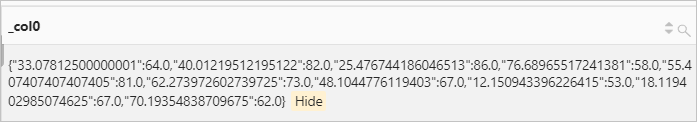• Example 2: Calculate the product of each request_time value and the weight of the value, group the values based on the calculated products, and then compute the approximate histogram of the request duration for the POST method.
• Query statement
``request_method:POST| SELECT numeric_histogram(10, request_time,case when request_time<20 then 100 else 10 end)``
• Query and analysis result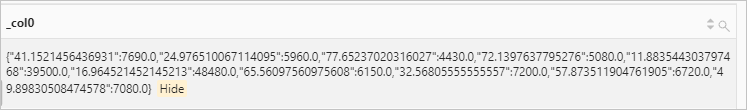## numeric_histogram_u function

The numeric_histogram_u function is used to compute the approximate histogram of x based on the number of histogram columns. The number is specified in the bucket parameter. A table that contains multiple rows and columns is returned.

### Syntax

``numeric_histogram_u(bucket, x)``

### Parameters

Parameter Description
bucket The number of columns in the histogram. The value of this parameter is of the bigint type.
x The value of this parameter is of the double type.

The double type.

### Examples

Compute the approximate histogram of the request duration for the POST method.

• Query statement
``request_method:POST | select numeric_histogram_u(10,request_time)``
• Query and analysis result